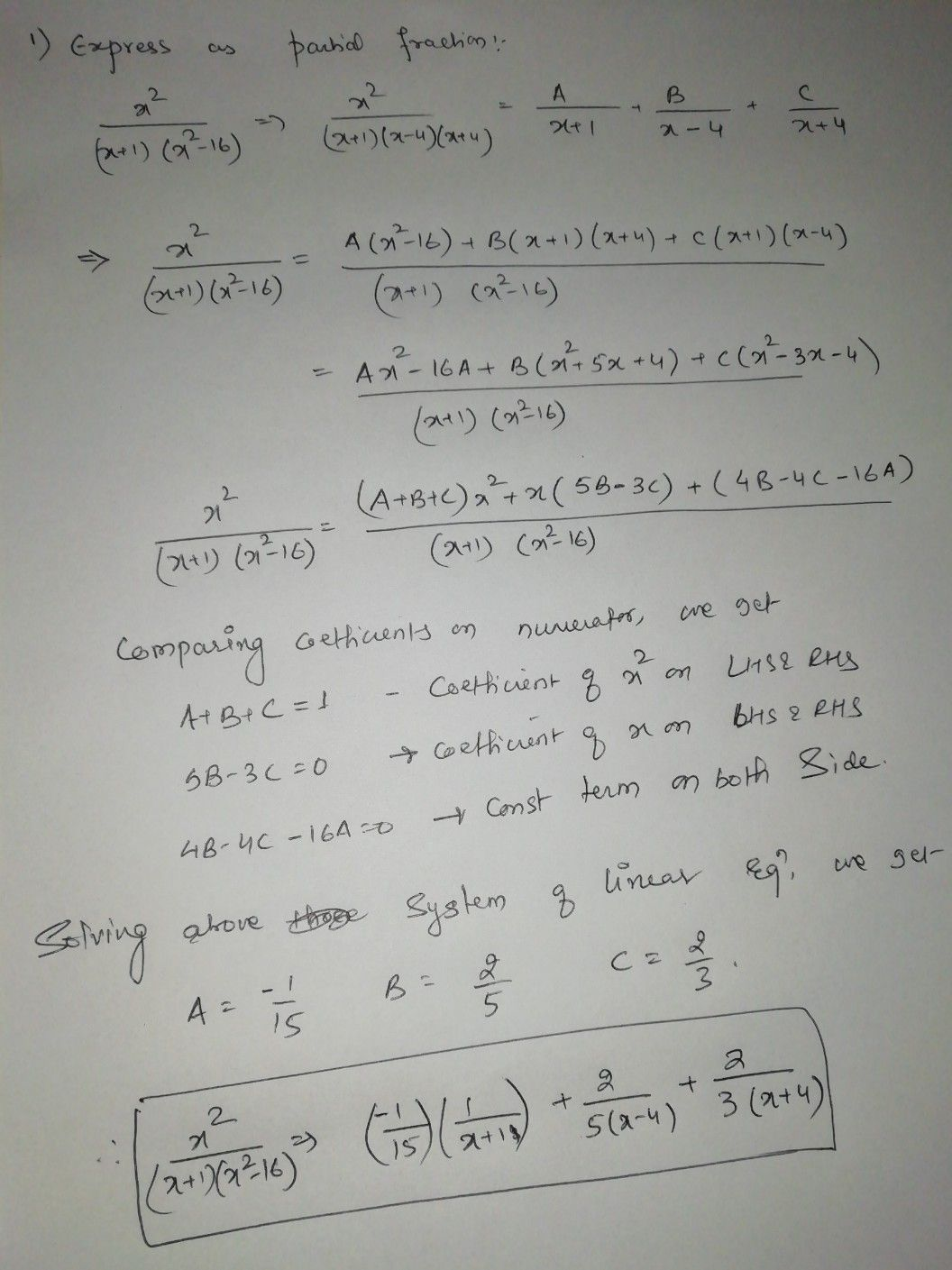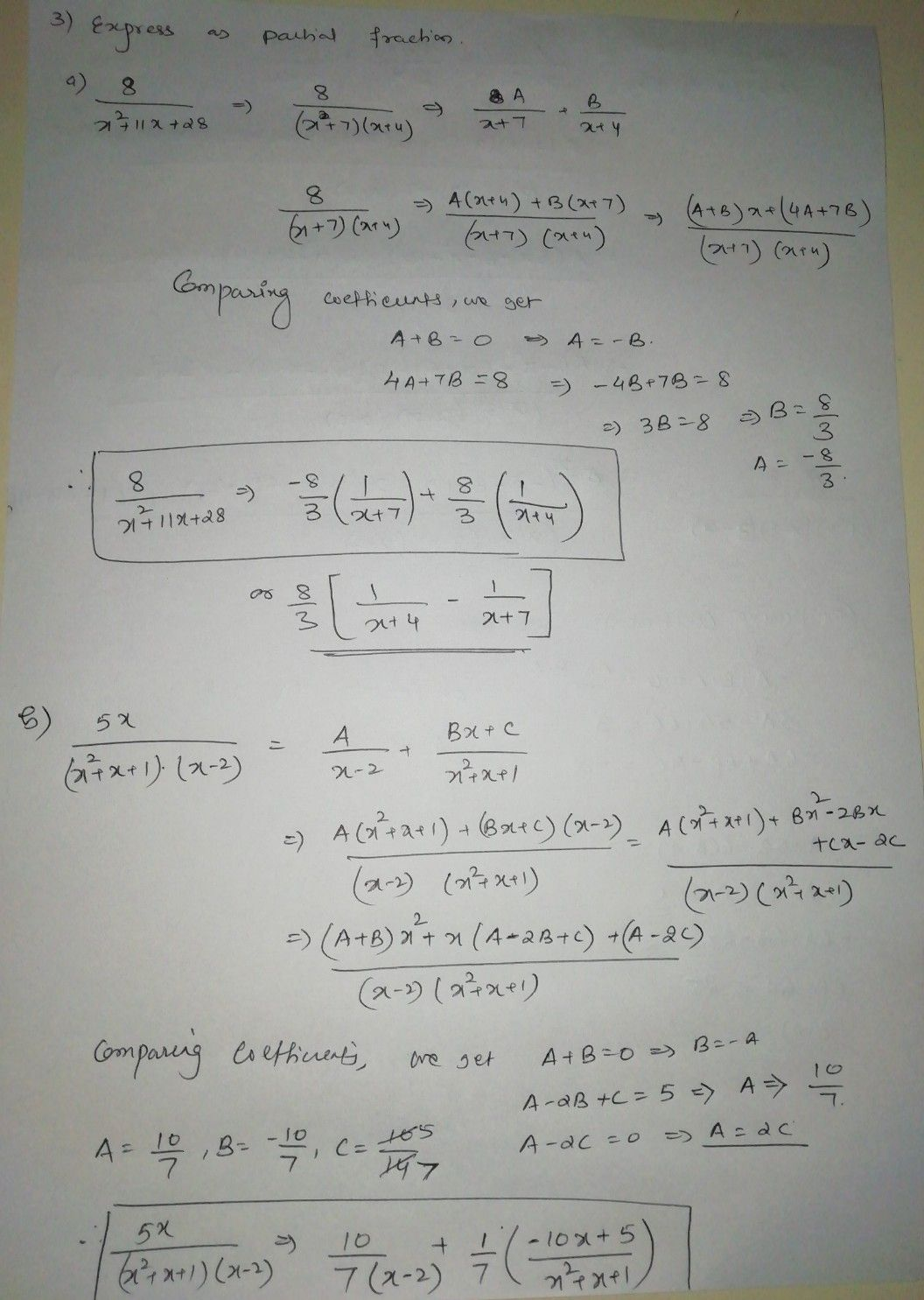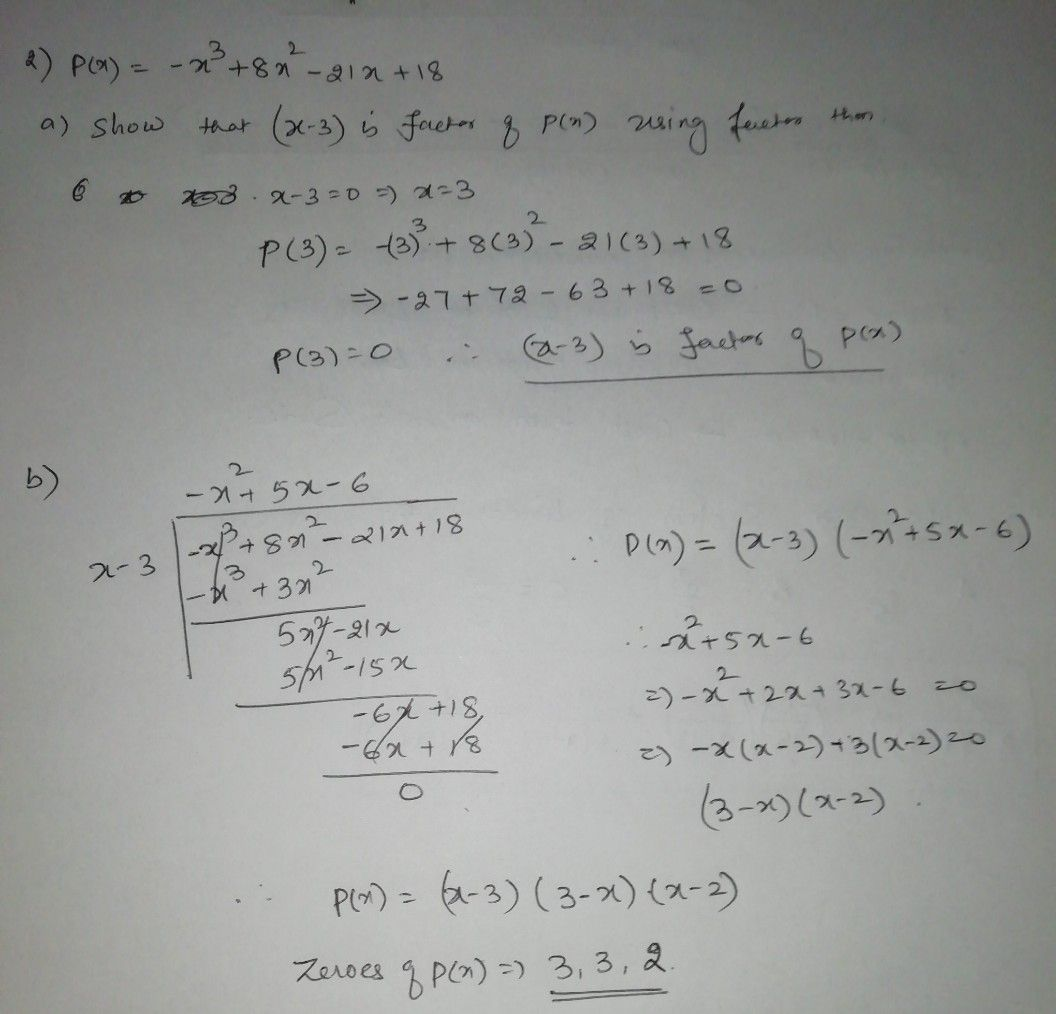Symbol
Problem$1$ Express $\dfrac {x^{2}} {\left(x+1\right)\left(x^{2}-16\right)}$ in partial fractions. $2$ Given polynomial $P\left(x\right)=-x^{3}+8x^{2}-21x+18$ $a\right)$ Show that $\left(x-3\right)$ is a factor of polynomial $P\left(x\right)=-x^{3}+8x^{2}-21x+18$ by using factor theorem. $b\right)$ Factorize P(x)completely by using long division and find all the zeroes. $c\right)$ Hence, express $\dfrac {3x-2} {P\left(x\right)}$ in partial fractions. $3$ Express the following as a sum of partial fractions. $8$ $a\right)$ $x^{2}+11x+28$ $5x$ $b\right)$ $\bar{\left(x^{2}+x+1\right)\left(x-2\right)}$